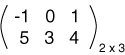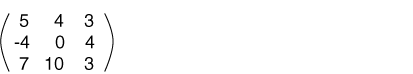What is the value of the element at row 3 column 1?

4

# Matrix Dimensions

The numbers of rows and columns of a matrix are called its dimensions. Here is a matrix with three rows and two columns:Sometimes the dimensions are written off to the side of the matrix, as in the above matrix. But this is just a little reminder and not actually part of the matrix. Here is a matrix with different dimensions. It has two rows and three columns. This is a different "data type" than the previous matrix.### QUESTION 3:

What do you suppose a square matrix is? Here is an example: